# Interior & Exterior Angles of a Triangle

Samuel Hoyland, Jeff Calareso
• Author
Samuel Hoyland

I have a bachelor's in math and music from Wesleyan University. I am currently pursuing a doctorate of music in composition at the University of Georgia. I have 9 years of work experience writing math content and 7 years of experience tutoring math one-on-one.

• Instructor
Jeff Calareso

Jeff teaches high school English, math and other subjects. He has a master's degree in writing and literature.

Understand the concept of interior angles and exterior angles. Learn how to measure the interior and exterior angles of a triangle through examples. Updated: 10/07/2021

Show

## What are Interior Angles?

A polygon is a closed two-dimensional shape made up of a number of straight line segments (called its sides). In a polygon, an interior angle is an angle that is inside the polygon. A triangle has three sides and three interior angles. In the triangle below, the three interior angles are labeled {eq}a {/eq}, {eq}b {/eq}, and {eq}c {/eq}.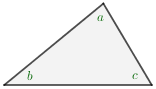Other polygons have more than three interior angles. The number of interior angles in a polygon is always equal to its number of sides. For instance, a hexagon like the one below has six sides and six interior angles.

## Missing Pieces

Did you ever work on a jigsaw puzzle, devoting hours and hours to putting it together, only to get almost to the end and find out a piece is missing? Maybe it's a piece you'd been looking for on and off for a while. 'There has to be a light blue sky piece somewhere here...'

When we're working with triangles, sometimes we have missing puzzle pieces. Here's an example: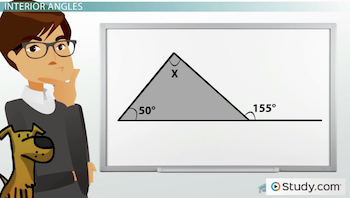We have a couple angles here, but what is X? How are we supposed to figure it out? Did we drop the answer on the floor? Did the dog eat it?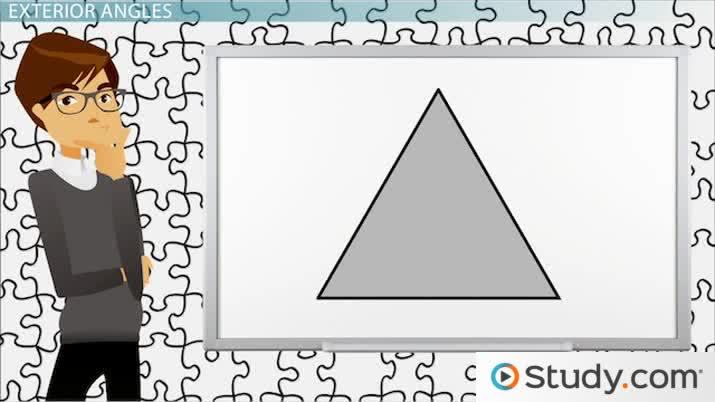An error occurred trying to load this video.

Try refreshing the page, or contact customer support.

Coming up next: Constructing the Median of a Triangle

### You're on a roll. Keep up the good work!

Replay
Your next lesson will play in 10 seconds
• 0:06 Missing Pieces
• 0:39 Interior Angles
• 2:01 Practice Problems
• 3:02 Exterior Angles
• 4:06 Practice Problems
• 4:50 Lesson Summary
Save Save

Want to watch this again later?

Timeline
Autoplay
Autoplay
Speed Speed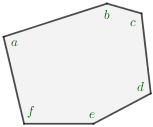## What are Exterior Angles?

To form an exterior angle, extend one of the sides past the angle. The angle formed from the extended side and the side that was next to (i.e. adjacent to) the original side is called an exterior angle. This angle will always be less than {eq}180^\circ {/eq}.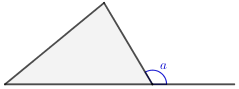A polygon with more than three sides also has exterior angles. For example, in the figure below, one exterior angle of a hexagon is labeled.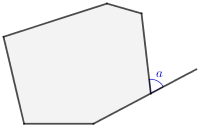## Interior vs. Exterior Angles

In the triangle below, an interior angle and an exterior angle that are adjacent are labeled {eq}a {/eq} and {eq}b {/eq}, respectively. Notice that these angles together form a straight angle (i.e. a straight line). Two adjacent angles that form a straight angle are called a linear pair. Since a straight angle measures {eq}180^\circ {/eq}, the angles in a linear pair always add up to {eq}180^\circ {/eq}. Angles that add up to {eq}180^\circ {/eq} (whether they are linear pairs or not) are called supplementary angles, so linear pairs are always supplementary angles.

For example, in the figure below, if {eq}a=58^\circ {/eq}, then {eq}b=180^\circ-58^\circ=122^\circ {/eq}.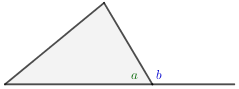In triangles and in polygons in general, there is a rule that pairs of adjacent interior and exterior angles will always be linear pairs and thus will always be supplementary.

## Interior and Exterior Angles of a Triangle

In polygons, the sum of the interior angles depends only on the number of sides (or the number of angles since they are always the same). For triangles, the sum of the interior angles will always be {eq}180^\circ {/eq}.

In the triangle below, we are given two of the three angles. We can find the missing angle by subtracting those two angles from {eq}180^\circ {/eq}. Thus, the missing third side measures {eq}180^\circ - 80^\circ - 70^\circ = 30^\circ {/eq}.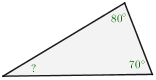In contrast, the sum of the exterior angles for all polygons, no matter the number of sides/angles, will always be {eq}360^\circ {/eq}. An example of this is shown in the hexagon below. Notice that the exterior angles of this hexagon add up to {eq}360^\circ {/eq}:

## Interior Angles

First, we should define what X is. If you're looking for a missing puzzle piece, you need to know what it is you need. X is an interior angle. An interior angle is an angle inside a shape. Since triangles have three angles, they have three interior angles.

In this triangle below, angles A, B and C are all interior angles.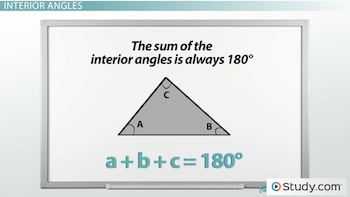Just as the pieces in a jigsaw puzzle fit together perfectly, the interior angles in a triangle must fit with each other.

The sum of the interior angles is always 180 degrees. In other words, a + b + c = 180 degrees.

Let's prove this. Below are two parallel lines. Let's add a triangle between them. At the top of our triangle, we have three angles based around our line. Let's label them X, Y and Z. These three angles form a straight line, so they add up to what? 180 degrees.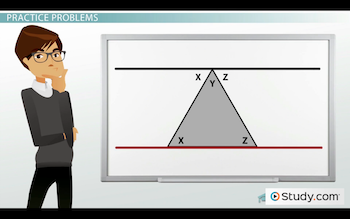Since we have a parallel line at the bottom of our triangle, we have alternate interior angles. So, the inside angle at the bottom is also equal to X. Z has an alternate interior angle at the bottom. And look what we did. We just proved that the sum of the interior angles of a triangle is 180 degrees.

## Practice Problems

Okay, so we know that. How can it help us? Remember our puzzle? In a triangle, you can never be stuck with one missing piece.

In the triangle we were just looking at above, what if we know that angle X is 35 degrees and angle Z is 60 degrees? Oh, man, if only we know angle Y, we'd know them all. But we do! It's 180 - 35 - 60, which is 85.

Here's another one: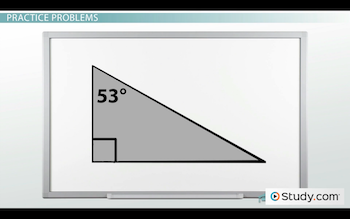In this one, we know angle X is 53 degrees. Wait, that's only one angle. We're losing pieces of this puzzle. Is it the cat? Is the cat stealing pieces? No. Look. See this symbol at the bottom left? This means that this angle is 90 degrees. So, we know we have a 53 degree angle and a 90 degree angle. If we subtract 53 and 90 from 180, we get 37 degrees. So, angle Y must be 37 degrees. And we can leave the cat alone.

To unlock this lesson you must be a Study.com Member.

Video Transcript

## Missing Pieces

Did you ever work on a jigsaw puzzle, devoting hours and hours to putting it together, only to get almost to the end and find out a piece is missing? Maybe it's a piece you'd been looking for on and off for a while. 'There has to be a light blue sky piece somewhere here...'

When we're working with triangles, sometimes we have missing puzzle pieces. Here's an example:We have a couple angles here, but what is X? How are we supposed to figure it out? Did we drop the answer on the floor? Did the dog eat it?

## Interior Angles

First, we should define what X is. If you're looking for a missing puzzle piece, you need to know what it is you need. X is an interior angle. An interior angle is an angle inside a shape. Since triangles have three angles, they have three interior angles.

In this triangle below, angles A, B and C are all interior angles.Just as the pieces in a jigsaw puzzle fit together perfectly, the interior angles in a triangle must fit with each other.

The sum of the interior angles is always 180 degrees. In other words, a + b + c = 180 degrees.

Let's prove this. Below are two parallel lines. Let's add a triangle between them. At the top of our triangle, we have three angles based around our line. Let's label them X, Y and Z. These three angles form a straight line, so they add up to what? 180 degrees.Since we have a parallel line at the bottom of our triangle, we have alternate interior angles. So, the inside angle at the bottom is also equal to X. Z has an alternate interior angle at the bottom. And look what we did. We just proved that the sum of the interior angles of a triangle is 180 degrees.

## Practice Problems

Okay, so we know that. How can it help us? Remember our puzzle? In a triangle, you can never be stuck with one missing piece.

In the triangle we were just looking at above, what if we know that angle X is 35 degrees and angle Z is 60 degrees? Oh, man, if only we know angle Y, we'd know them all. But we do! It's 180 - 35 - 60, which is 85.

Here's another one:In this one, we know angle X is 53 degrees. Wait, that's only one angle. We're losing pieces of this puzzle. Is it the cat? Is the cat stealing pieces? No. Look. See this symbol at the bottom left? This means that this angle is 90 degrees. So, we know we have a 53 degree angle and a 90 degree angle. If we subtract 53 and 90 from 180, we get 37 degrees. So, angle Y must be 37 degrees. And we can leave the cat alone.

To unlock this lesson you must be a Study.com Member.

#### Do exterior angles add up to 360?

Yes, the sum of the exterior angles for all polygons, no matter the number of sides/angles, will always be 360 degrees.

#### What is interior angle with example?

In a polygon, an interior angle is an angle that is inside the polygon. For instance, a triangle has three interior angles and a hexagon has six interior angles.

#### What is an example of an exterior angle?

To form an exterior angle, extend one of the sides past the angle. The angle that is formed from the extended side and the adjacent side is called an exterior angle.

#### How do you find the exterior angle?

Adjacent exterior and interior angles always add up to 180 degrees (i.e. are supplementary angles). That means you can find the exterior angle by subtracting the interior angle from 180 degrees.

### Register to view this lesson

Are you a student or a teacher?

Back

### Resources created by teachers for teachers

Over 30,000 video lessons & teaching resources‐all in one place.Video lessonsQuizzes & WorksheetsClassroom IntegrationLesson Plans

I would definitely recommend Study.com to my colleagues. It’s like a teacher waved a magic wand and did the work for me. I feel like it’s a lifeline.

Jennifer B.
TeacherCreate an account to start this course today
Used by over 30 million students worldwide